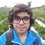# Pre-RMO 2014/6

What is the smallest possible natural number $n$ for which the equation $x^2 - nx + 2014 = 0$ has integer roots?

This note is part of the set Pre-RMO 2014Note by Pranshu Gaba
6 years, 9 months ago

This discussion board is a place to discuss our Daily Challenges and the math and science related to those challenges. Explanations are more than just a solution — they should explain the steps and thinking strategies that you used to obtain the solution. Comments should further the discussion of math and science.

When posting on Brilliant:

• Use the emojis to react to an explanation, whether you're congratulating a job well done , or just really confused .
• Ask specific questions about the challenge or the steps in somebody's explanation. Well-posed questions can add a lot to the discussion, but posting "I don't understand!" doesn't help anyone.
• Try to contribute something new to the discussion, whether it is an extension, generalization or other idea related to the challenge.

MarkdownAppears as
*italics* or _italics_ italics
**bold** or __bold__ bold
- bulleted- list
• bulleted
• list
1. numbered2. list
1. numbered
2. list
Note: you must add a full line of space before and after lists for them to show up correctly
paragraph 1paragraph 2

paragraph 1

paragraph 2

[example link](https://brilliant.org)example link
> This is a quote
This is a quote
    # I indented these lines
# 4 spaces, and now they show
# up as a code block.

print "hello world"
# I indented these lines
# 4 spaces, and now they show
# up as a code block.

print "hello world"
MathAppears as
Remember to wrap math in $$ ... $$ or $ ... $ to ensure proper formatting.
2 \times 3 $2 \times 3$
2^{34} $2^{34}$
a_{i-1} $a_{i-1}$
\frac{2}{3} $\frac{2}{3}$
\sqrt{2} $\sqrt{2}$
\sum_{i=1}^3 $\sum_{i=1}^3$
\sin \theta $\sin \theta$
\boxed{123} $\boxed{123}$

Sort by:

Let the roots of the equation be $\alpha$ and $\beta$.

Then $n = \alpha + \beta$ and $2014 = \alpha \beta$.

The possible integral pairs of $(\alpha, \beta)$ are $(1, 2014), (2, 1007), (19, 106),$ and $(38, 53)$.

Therefore, the possible values of $n$ are $2015, 1009, 125$ and $91$. The minimum value is $91$, so $n = \boxed{91}$

- 6 years, 9 months ago

- 6 years, 9 months ago

Yes , it is 91 :D

- 6 years, 9 months ago

Yayy!! lol:D:D

- 6 years, 9 months ago

Explain?

- 6 years, 9 months ago

The discriminant of the above equation should be a perfect square for the equation to have integral roots. So the smallest value of n for which it is a perfect square is 91.

- 6 years, 9 months ago

91

- 6 years, 3 months ago

91

- 5 years, 11 months ago

91

- 5 years, 11 months ago